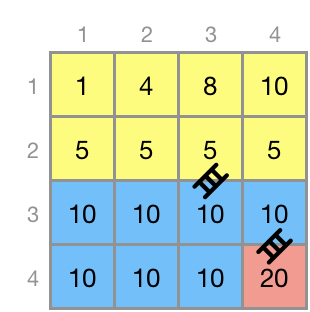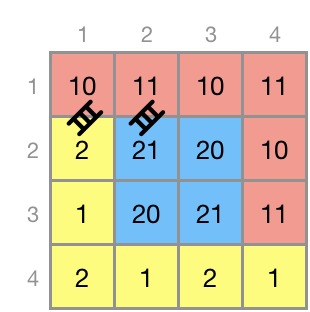###### Description

There is an N x N sized square grid. Each grid cell is 1 x 1 size, with one number printed inside. The numbers in the grid indicate the height of the cell.

Start from any cell of this grid, we are going to visit all the squares. When moving a cell, you can move it up, down, left, and right by one cell. The height difference between the current cell and the cell you want to move must be less than the given `height`. At this time, installing the ladder costs as much as the height difference between the two cells. Therefore, we should minimize the cost of installing ladders so that it can be moved to all the spaces with the lowest possible cost. There is no limit to the number of ladders, and the installed ladders will not be removed.

When a 2-dimensional array `land` containing the height of each grid cell and the maximum height of the movable `height` are given as parameters, complete the solution function to return the minimum value of the ladder installation cost required to visit all cells.

##### Constraints
• `land` is an N x N sized square grid.
• The minimum size of `land` is 4 x 4, and maximum 300 x 300.
• The elements of `land` represent the height of each grid cell.
• The height of the grid is a natural number between 1 and 10,000 inclusive.
• `height` is a natural number between 1 and 10,000 inclusive.
##### Examples
land height result
[[1, 4, 8, 10], [5, 5, 5, 5], [10, 10, 10, 10], [10, 10, 10, 20]] 3 15
[[10, 11, 10, 11], [2, 21, 20, 10], [1, 20, 21, 11], [2, 1, 2, 1]] 1 18

Example #1

The height of each cell is as follows. If the height difference is less than 3, it is possible to move without a ladder.In the above image, the movable range without using the ladder is painted with the same color. For example, you can not move directly from a 4-height cell (row 1, column 3) to an 8-height column. But if you go through the cell height of 5, it is possible without a ladder.

So if you install just two ladders like above, you can visit all the cells and the minimum cost is 15.

• Cell height 5 → Cell height 10 : costs 5
• Cell height 10 → Cell height 20 : costs 10

Example #2

The height of each cell is as follows. If the height difference is less than 1, it is possible to move without a ladder.If ladders are installed at (row 2, column 1) → (row 1, column 1) and (row 1, column 2) → (row 2, column 2) as above, it costs 18 and this is the minimum.

Result
Result of [Run Test] or [Submit] will be displayed here